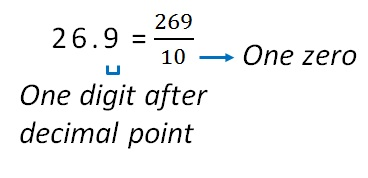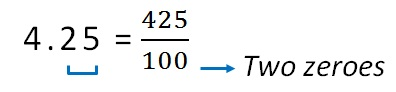Decimals to fractions

Chapter 8 Class 6 Decimals
Concept wise

Let’s do this by an example

46.8

There is only one digit after decimal point,

If we remove decimal point, we divide by 10

46.8 = 468/10

Similarly,And,

428.95 = 42895/100

26.5 = 265/10

89.8 = 898/10

Learn in your speed, with individual attention - Teachoo Maths 1-on-1 Class

### Transcript

Two digits after decimal point And, 428.95 = 42895/100 26.5 = 265/10 89.8 = 898/10